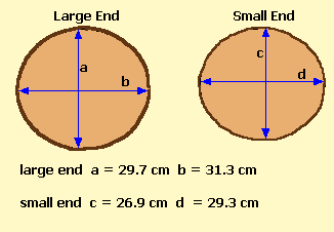Spike's Calculators

# William KlemmeKlemme calculates the volume of a log in cubic meters and is used to scale logs for export to the People's Republic of China.

Average diameters have to be calculated from both ends of the log.
Diameters measured to the nearest mm need to be rounded to the nearest whole centimetre.for the large end 29.7 + 31.3 = 61 / 2 = 31.5 cm enter as 32 for large end diameter
for the small end 26.9 + 29.3 = 56.2 /2 = 28.1 cm enter as 28 for the small end diameter
Diameter Measured Inside Bark = DIB